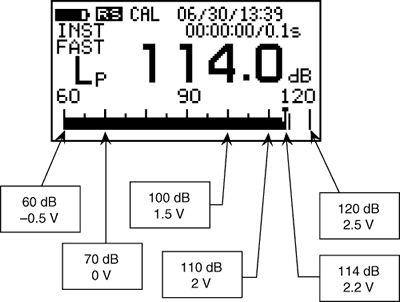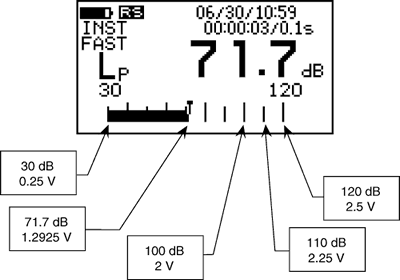Sound Level Meter FAQDC output1. DC output in normal range

The specifications of DC output are as follows;

• Full scale output level 2.5 V ±10 mV

• Scale factor 0.5 V ±10 mV/10 dB

Therefore, when the steady sine wave is input, the DC output at the upper limit for the bar indicator is 2.5 V. The scale factor is 0.5 V/10 dB. When the measurement level of the steady signal is adB above the upper limit of the bar indicator, the output is expressed as follows;

2.5+0.5×a/10 V

For example, when the measurement level of the steady signal is -20 dB above the upper limit of the bar indicator, the output is expressed as follows; (When the level range is 60 to 120 dB, the measurement level is equivalent to 100 dB. When the level range is 40 to 120 dB in real-time mode, it is equivalent to 100 dB.)

2.5+0.5×(-20)/10 = 1.5 V

As the electric calibration signal is -6 dB above the upper limit of the bar indicator. The DC output is;

2.5+0.5×(-6)/10 = 2.2 V

(When the level range is 60 to 120 dB, the measurement level is equivalent to 114 dB.) When the DC output level is aV, the indicated value is separated from the upper limit of the bar indicator by

(a-2.5)/0.5×10 dB

For example, when the DC output is 1.65 V, the indicated value is separated from the full scale by

(1.65-2.5)/0.5×10 = -17 dB

When the level range is 60 to 120 dB, the measurement level is equivalent to 103 dB. When the level range is 40 to 120 dB in real-time mode, it is equivalent to 103 dB. The maximum DC output level is 3 V which is the same as the level of sine wave indicating 10 dB greater than the full scale. If the DC output level exceeds this value, the over mark is displayed.2. DC output in wide range

The specifications of the DC output are as follows;

• Full scale output level 2.5 V ±5 mV

• Scale factor 0.25 V ±5 mV/10 dB

Therefore, when a steady sine wave is input, the DC output at the upper limit for the bar indicator is 2.5 V. The scale factor is 0.52 V/10 dB. When the measurement level of the steady signal is adB above the upper limit of the bar indicator, the output is expressed as follows;

2.5+0.25×a/10 V

For example, when the measurement level of the steady signal is -20 dB above the upper limit of the bar indicator, the output is expressed as follows;

2.5+0.25×(-20)/10 = 2 V

As the electrical calibration signal is -16 dB above the upper limit of the bar indicator. The DC output is

2.5+0.25×(-16)/10 = 2.1 V

(When the level range is 30 to 120 dB, the measurement level is equivalent to 104 dB.) When the DC output level is aV, the indicated value is separated from the upper limit of the bar indicator by

(a-2.5)/0.25×10 dB

For example, when the DC output is 1.295 V, the indicated value is separated from the full scale by

(1.2925-2.5)/0.25×10 = -48.3 dB

When the level range is 30 to 120 dB, the measurement level is equivalent to 71.7 dB. The maximum DC output level is +2.75 V which is the same as the level of sine wave indicating 10 dB greater than the full scale. If the DC output level exceeds this value, the over mark is displayed.Revised:2001.10.12• Copyright © ONO SOKKI CO.,LTD All Rights Reserved.